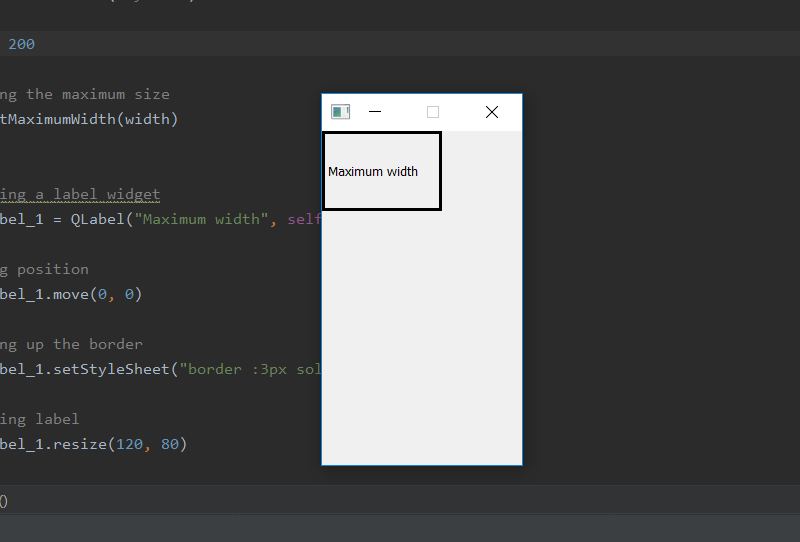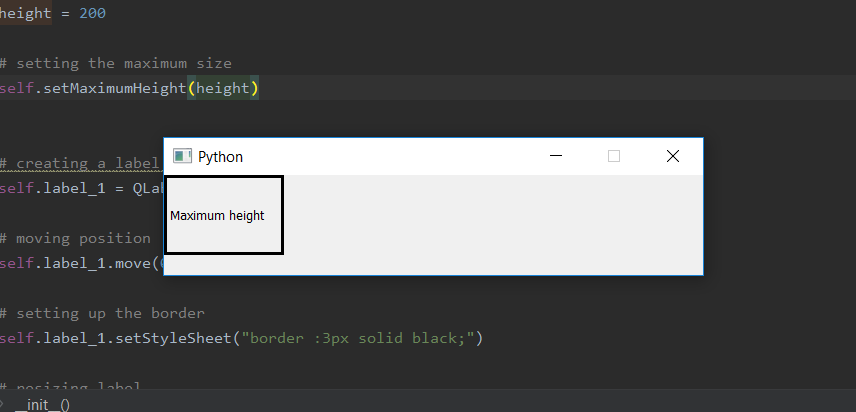# PyQt5 – Set maximum size for width or height of window

When we create a window, by default the window size is resizable, although we can use `setMaximumSize()` method to set the maximum size of the window. But what if we want to set maximum length only for width or height only. In order to do so we use `setMaximumWidth()` and `setMaximumHeight()` method to set maximum width / height. When we use these method other length will be variable i.e there will be no maximum length to it, it can be stretched to the size of screen.

Syntax :

```self.setMaximumWidth(width)
self.setMaximumHeight(height)
```

Argument : Both takes integer as argument.

Action performed :
`setMaximumWidth()` sets the maximum width.
`setMaximumHeight()` sets the maximum height.

Code for maximum width –

 `# importing the required libraries ` ` `  `from` `PyQt5.QtCore ``import` `*`  `from` `PyQt5.QtGui ``import` `*`  `from` `PyQt5.QtWidgets ``import` `*`  `import` `sys ` ` `  ` `  `class` `Window(QMainWindow): ` `    ``def` `__init__(``self``): ` `        ``super``().__init__() ` ` `  `        ``# set the title ` `        ``self``.setWindowTitle(``"Python"``) ` ` `  `        ``width ``=` `200` ` `  `        ``# setting the maximum width ` `        ``self``.setMaximumWidth(width) ` ` `  `        ``# creating a label widget ` `        ``self``.label_1 ``=` `QLabel(``"Maximum width"``, ``self``) ` ` `  `        ``# moving position ` `        ``self``.label_1.move(``0``, ``0``) ` ` `  `        ``# setting up the border ` `        ``self``.label_1.setStyleSheet(``"border :3px solid black;"``) ` ` `  `        ``# resizing label ` `        ``self``.label_1.resize(``120``, ``80``) ` ` `  `        ``# show all the widgets ` `        ``self``.show() ` ` `  ` `  `# create pyqt5 app ` `App ``=` `QApplication(sys.argv) ` ` `  `# create the instance of our Window ` `window ``=` `Window() ` ` `  `# start the app ` `sys.exit(App.``exec``()) `

Output :Code for maximum height –

 `# importing the required libraries ` ` `  `from` `PyQt5.QtCore ``import` `*`  `from` `PyQt5.QtGui ``import` `*`  `from` `PyQt5.QtWidgets ``import` `*`  `import` `sys ` ` `  ` `  `class` `Window(QMainWindow): ` `    ``def` `__init__(``self``): ` `        ``super``().__init__() ` ` `  ` `  `        ``# set the title ` `        ``self``.setWindowTitle(``"Python"``) ` ` `  `        ``height ``=` `200` ` `  `        ``# setting the maximum height ` `        ``self``.setMaximumHeight(height) ` ` `  ` `  `        ``# creating a label widget ` `        ``self``.label_1 ``=` `QLabel(``"Maximum height"``, ``self``) ` ` `  `        ``# moving position ` `        ``self``.label_1.move(``0``, ``0``) ` ` `  `        ``# setting up the border ` `        ``self``.label_1.setStyleSheet(``"border :3px solid black;"``) ` ` `  `        ``# resizing label ` `        ``self``.label_1.resize(``120``, ``80``) ` ` `  `        ``# show all the widgets ` `        ``self``.show() ` ` `  ` `  `# create pyqt5 app ` `App ``=` `QApplication(sys.argv) ` ` `  `# create the instance of our Window ` `window ``=` `Window() ` ` `  `# start the app ` `sys.exit(App.``exec``()) `

Output :My Personal Notes arrow_drop_upCheck out this Author's contributed articles.

If you like GeeksforGeeks and would like to contribute, you can also write an article using contribute.geeksforgeeks.org or mail your article to contribute@geeksforgeeks.org. See your article appearing on the GeeksforGeeks main page and help other Geeks.

Please Improve this article if you find anything incorrect by clicking on the "Improve Article" button below.

Article Tags :

Be the First to upvote.

Please write to us at contribute@geeksforgeeks.org to report any issue with the above content.